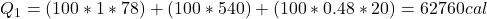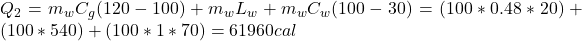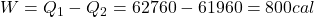## We are going to make an imaginary engine using water. We are going to heat 100 grams of water to 120 C from its initial temperature of 22 C.

Question

We are going to make an imaginary engine using water. We are going to heat 100 grams of water to 120 C from its initial temperature of 22 C. This would be the heat extracted from the hot reservoir. Then the steam will condense back into water and will be at a temperature of 30 C. This would be the exhaust expelled into the cold reservoir. You can assume the mass of the water and the mass of the steam is the same in this case. How much work can be done using this engine? The specific heat of water is 1 cal/(g C), the specific heat of steam is 0.48 cal/(g C),
and the latent heat of vaporization 540 cal/g.

in progress 0
6 months 2021-07-29T20:33:08+00:00 1 Answers 2 views 0

The work done by this engine is 800 cal

Explanation:

Given:

100 g of water

120°C final temperature

22°C initial temperature

30°C is the temperature of condensed steam

Cw = specific heat of water = 1 cal/g °C

Cg = specific heat of steam = 0.48 cal/g °C

Lw = latent heat of vaporization = 540 cal/g

Question: How much work can be done using this engine, W = ?

First, you need to calculate the heat that it is necessary to change water to steam:Here, mw is the mass of waterNow, you need to calculate the heat released by the steam:The work done by this engine is the difference between both heats: